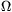Mathematical and Physical Journal
for High Schools
Issued by the MATFUND Foundation
 Already signed up? New to KöMaL?

#Problem P. 4033. (January 2008)

P. 4033. Three cells are connected in series. Their electromotive forces are 2 V, 10 V, and 5 V; their internal resistances are 0.2, 0.3, and 0.5. Calculate the voltage across the third cell and graph this terminal voltage as a function of the external resistance connected to the circuit.

(4 pont)

Deadline expired on February 11, 2008.

### Statistics:

 87 students sent a solution. 4 points: 54 students. 3 points: 18 students. 2 points: 10 students. 0 point: 2 students. Unfair, not evaluated: 3 solutionss.

Problems in Physics of KöMaL, January 2008